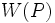# Weakly closed conjugacy functor

(diff) ← Older revision | Latest revision (diff) | Newer revision → (diff)
Suppose$G$ is a finite group,$p$ a prime number, and$W$ a conjugacy functor on$G$ with respect to$p$. We say that$W$ is weakly closed in$G$ with respect to$p$ if$W(P)$ is a weakly closed subgroup of$P$.